## Saturday, 15 December 2012

### CBSE Class 10 - Physics - CH11: The Human Eye and the Colourful World (NCERT Q & A)

The Human Eye and the Colourful World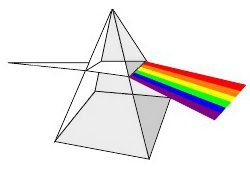Dispersion of Light Which light bends the most? credits:wpclipart
(NCERT Chapter Q & A, Other Questions asked in CBSE Exam)

Q1(NCERT): What is meant by power of accommodation of the eye?

Answer: Power of accommodation is the ability of the eye lens to focus near and far objects clearly on the retina by adjusting its focal length. Power of accommodation of the eye is limited. It implies the focal length of the eye lens cannot be reduced beyond certain minimum limit.

Q2(CBSE 2011): A person cannot see the objects distinctly, when placed at a distance less than 50 cm.
(a) Identify the defect of vision.
(b) Give two reasons for this defect.
(c) Calculate the power and nature of the lens he should be using to see clearly the object placed at a distance of 25 cm from his eyes.
(d) Draw the ray diagrams for the defective and the corrected eye.

Answer: (a) Defect of vision = Hypermetropia  or Long-sightedness

(b)  Reasons for the defect are:
• Curvature of lens or retina becomes less than normal increases focal length.
• Ciliary muscles become stiff
• Shortening of eye ball due to which distance between retina and and lens reduces and image is formed beyond retina.
(c) Given, u = -25 cm, v = -50cm,  f = ?
Applying lens formula,      1/f = 1/v - 1/u
⇒1/f = -1/50 - (-1/25) = -1/50 + 1/25 = (-1+2) /50 = 1/50
⇒ f = 50cm = 0.5m
∴ Power of the lens = 1/f = 1/0.5m = +2D
∴ A convex lens having power of 2D can be used to correct the vision.

(d) ray diagrams for the defective and the corrected eye.

Q3(NCERT): A person with a myopic eye cannot see objects beyond 1.2 m distinctly. What should be the type of the corrective lens used to restore proper vision?

Answer: The distance of far point x = 1.2m
To view distant objects, concave lens of focal length -1.2 should be used.
i.e. f = -x = -1.2m
Power of lens = 1/f = 1/(-1.2m) = -0.83D

Q4(CBSE 2011): Name the part of the human eye that helps in changing the focal length of the eye lens.

Answer: Ciliary muscles.
(Note: Pupil regulates and controls the amount of light entering the eye.)

Q5(NCERT MCQ): The human eye can focus objects at different distances by adjusting the focal length of the eye lens. This is due to

(a) presbyopia.
(b) accommodation.
(c) near-sightedness.
(d) far-sightedness.

Answer: (b) accommodation.

Q6(NCERT MCQ): The human eye forms the image of an object at its
(a) cornea.
(b) iris.
(c) pupil.
(d) retina.

Answer:  (d) retina.

Q7(NCERT MCQ): The least distance of distinct vision for a young adult with normal vision is about
(a) 25 m.
(b) 2.5 cm.
(c) 25 cm.
(d) 2.5 m.

Answer: 25cm

Q8(NCERT MCQ): The change in focal length of an eye lens is caused by the action of the
(a) pupil.
(b) retina.
(c) ciliary muscles.
(d) iris.

Answer: (c) ciliary muscles.

Q9(NCERT): What is the far point and near point of the human eye with normal vision?

Answer: The near point of the eye is the minimum distance of the object from the eye, which
can be seen clearly. For a normal human eye, this distance is 25cm.

The far point of the eye is the maximum distance upto which the eye can see the objects clearly without strain. The far point of the normal human eye is infinity.

Q10(CBSE 2011): A boy uses spectacles of focal length – 60 cm. Name the defect of vision he is suffering from. Which lens is used for the correction of this defect? Compute the power of this lens.

Answer:  Given f = -60 cm =-0.60m
The -ve sign of focal length indicates that the lens is concave.  ∴ the boy suffers from myopic (short sightedness) vision.
Power of the lens = 1/f(in m) = 1/(-0.60) = -1.67 D

Q11(NCERT): A student has difficulty reading the blackboard while sitting in the last row. What could be the defect the child is suffering from? How can it be corrected?

Answer: The student has difficulty in seeing distant objects. He might be suffering from myopia. The defect can be corrected using a concave lens of appropriate focal length.Myopic Eye Correction using Concave lens image credits:wikipedia

Q12(CBSE): Why do we have two eyes instead of one?

Answer: Having two eyes instead of one is advantageous for the following:
• field of view is more with two eyes than one eye.
• two eyes gives us three dimensional vision (stereo vision) of an object.

Q13(NCERT): A person needs a lens of power –5.5 dioptres for correcting his distant vision. For correcting his near vision he needs a lens of power +1.5 dioptre. What is the focal length of the lens required for correcting (i) distant vision, and (ii) near vision?

Answer:
(i) Distant vision:  Given focal length (f) = ?, Power (P) = -5.5D
P = 1/f  ⇒ f = 1/P = 1/(-5.5)m = -100/55cm = -18.2 cm

(ii) For near vision, P = +1.5 D
⇒ f = 1/P = 1/1.5m = 1000/15cm = 66.7 cm

Q14(CBSE 2012): Define dispersion of white light and name the colours of white light in order?

Answer: The splitting of light into its component colours is called dispersion. White light splits into seven colours (VIBGYOR) i.e. violet, indigo, blue, green, yellow, orange and red. Isaac Newton was the first to use a glass prism to obtain the spectrum of sunlight.

Q15(NCERT): The far point of a myopic person is 80 cm in front of the eye. What is the nature and power of the lens required to correct the problem?

Answer:  Given, distance of far point (x) = 80 cm, P = ?

To view distant objects correctly, focal length of the corrective lens = f = -x = -80cm

Power of the lens (P = 1/(f in metres) = 1/(-0.80) = 100/-80 = -1.25D
The lens is concave of powed -1.25D

Q16(NCERT): Make a diagram to show how hypermetropia is corrected. The near point of a hypermetropic eye is 1 m. What is the power of the lens required to correct this defect? Assume that the near point of the normal eye is 25 cm.

Answer: For diagram see question 2(b) above.
Given  v = -1m = -100cm
u = -25cm
Using lens formula,   1/v -/1u = 1/f
⇒ -1/100 + 1/25 = 1/f
⇒ f = 33.3 cm  - 0.333m

Power of the lens = 1/f  = 100/33.3 = 3D

Q17(NCERT): Why is a normal eye not able to see clearly the objects placed closer than 25 cm?

Answer: Since the focal length of eye lens cannot be decreased beyond certain minimum length.

Q18(Old CBSE Curr. 2006): List four common defects which can be corrected with the use of spectacles.

Answer: Four common defects that can be corrected by using spectacles:
1. Hypermetropia
2. Myopia
3. Astigmatism
4. Presbyopia

Q19(NCERT): What happens to the image distance in the eye when we increase the distance of an object from the eye?

Answer: The size of the eye can change, so the image distance is fixed. When we increase the distance of an object from the eye, the image distance in the eye does not change. Due to power of accommodation of the eye, focal length of the eye lens is changed which compensates the increase in object distance. Hence image distance remains fixed and image is formed on the retina of the eye.

Q20(NCERT): Why do stars twinkle?
OR
Q(CBSE 2012): Explain why do stars twinkle and planets do not?

Answer: The twinkling of a star is due to the earth's atmospheric refraction. The earth's atmosphere is moving and it consists of pockets of warm and cold air. Thus the atmosphere has variations in refractive indices of air. Stars are very far away from the earth and emit their own light. Being very far from the earth, these are considered point sources. When a star light enters the earth's atmosphere, it undergoes multiple refractions and bends continuously towards the normal till it enters our eyes. Its apparent position appears higher than the normal one. Due to mobility of air and variation is temperature, this apparent position of star is not steady and moves continuously giving a twinkling effect.
When in space (outside the earth's atmosphere) stars do not appear twinkle. Planets as compared to stars are closer to the earth and appear bigger (we cannot consider them point sized like stars). It nullifies the twinkling effect.

Q21(NCERT): Explain why the planets do not twinkle.

Answer:  The distanced between planets and the earth is less as compared to stars. Planets cannot be considered as point sources. The apparent shift in their position due to their position cannot be observed because they subtend greater angle at the eye. Comparatively larger size, their brightness do not change and do not give twinkle effect.

Q22: What is Mirage? How does it occur?Mirage (1-Tree, 2 - cold and dense layer, 3 - Tree image, 4- hot layer of air, 5 - eye)

Answer: A mirage is an optical illusion which is generally observed in deserts or over hot surfaces like a coal tarred road during hot summers. In summers, the layer near to earth surface is hotter and lighter than upper layers of the air. Due to variation of optical densities of air layers, light from an object (e.g. tree) undergoes series of internal reflections and bend upwards. It gives an inverted virtual image below the seen object. To a distant traveller it gives shinning water effect called mirage.Mirage (credits:wikipedia)

Q23: What are the causes of myopia?

Answer: Causes of  myopia are:
• Increase in the length of the eye ball leads to increase in distance of the retina from the eye.
• Decrease in focal length of eye lens.

Q24: What is Tyndall effect?

Answer: The scattering of light by colloidal particles is called Tyndall effect. When a beam of light strikes the minute particles of earth’s atmosphere which are suspended particles of dust and molecule of air the path of beam become visible. The phenomenon of scattering of light by the colloidal particle is called Tyndall Effect. It can be observed when sunlight passes through a canopy of a dense forest.

Q25: How does colour of scattered light depend on colloidal particles?

Answer: The colour of the scattered light depends on the size of the scattering particles (as shown below).

(In progress...)#### 26 comments:

1.this is useless

2.its just waste of time such a waste blogspot has been created

3.its just waste of time such a waste blogspot has been created

4.this is useless

5.Nice article, can tell you put a lot of hard work into it :)

6.This comment has been removed by the author.

7.8.9.Thank you so much. :)

10.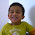good ...but no explanation for important questions.....for kids such problems are good..😝😝

11.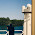if you didnt like this... then go head blame ncert and cbse
because these are from ncert text book and cbse papers.from exam point of view its pretty good

12.13.Too good !!

14.a nice one..but plz add few more complicated myopic question
and diff. question on dispersion n scattering of light

15.a nice one..but plz add few more complicated myopic question
and diff. question on dispersion n scattering of light

16.17.Bakwas
Ek dum bakwas
Stupid
Idiot
Nasty
Akward

1.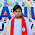Y???
U should not discourage the google, it supplies every thing

18.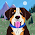thanks.....very helpful

19.WONDERFUL Q&A EDUCATING

20.answer 20:

Since the physical conditions of the Earth’s atmosphere keeps changing, the apparent position of the
star is not stationary. The star changes its position continuously, which makes it twinkle. This happens
because starlight travels a very large distance before reaching the observer. However, the path varies
continuously because of uneven atmospheric conditions. Hence, the stars seem to be fluctuating,
sometimes appearing brighter and sometimes fainter. All this together, gives rise to the twinkling
effect of stars.
The sun and the other planets of the solar system are relatively closer to the Earth. Thus, these are
not seen as point sources like stars, but are considered as extended sources. Any variation or
fluctuation of light coming from any part cancels out with each other. This results in zero fluctuation.
Hence, the sun and the planets do not twinkle.

21.Really it's not good

22.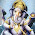23.very helpful

24.very helpful

25.Best notes and questions for competitive 10th level exams and board exams 5*

We love to hear your thoughts about this post!